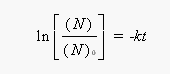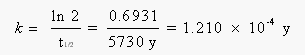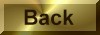Practice Problem 9

The skin, bones and clothing of an adult female mummy discovered in Chimney Cave, Lake Winnemucca, Nevada, were dated by radiocarbon analysis.  How old is this mummy if the sample retains 73.9% of the activity of living tissue?

Solution

Because 14C decays by first-order kinetics, the log of the ratio of the 14C in the sample today (N) to the amount that would be present if it was still alive (N0) is proportional to the rate constant for this decay and the time since death:The rate constant for this reaction can be calculated from the half-life of 14C, which is 5730 years:If the sample retains 73.9% of its activity, the ratio of the activity today (N) to the original activity (N0) is 0.739.  Substituting what we know into the integrated form of the first-order rate law gives the following equation:

ln (0.739) = -(1.210 x 10-4 y-1)(t)

Solving the equation for the unknown gives an estimate of the time since death:

t = 2500 years